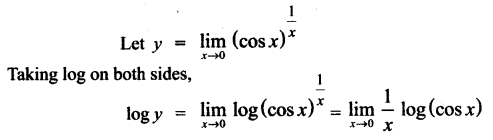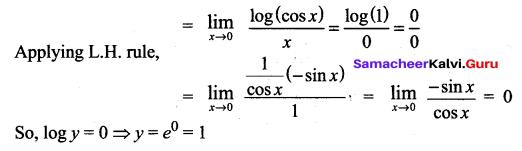# Samacheer Kalvi 12th Maths Solutions Chapter 7 Applications of Differential Calculus Ex 7.5

### Tamilnadu Samacheer Kalvi 12th Maths Solutions Chapter 7 Applications of Differential Calculus Ex 7.5

Evaluate the following limits, if necessary use l’Hopital Rule.

Question 1.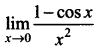Solution: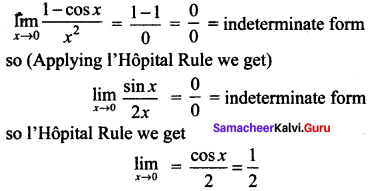Question 2.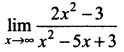Solution: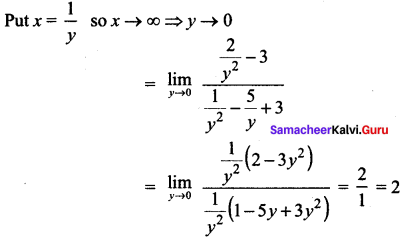Question 3.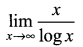Solution: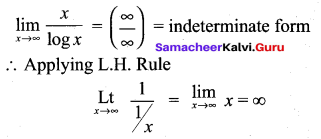Question 4.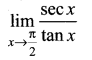Solution: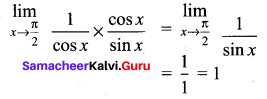Question 5.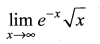Solution: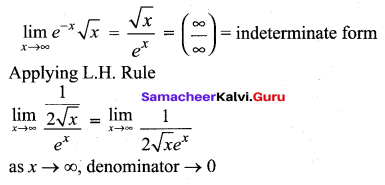Question 6.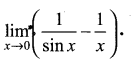Solution: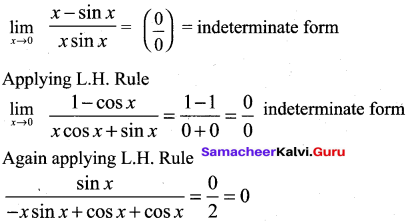Question 7.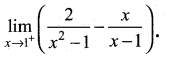Solution: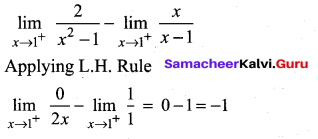Question 8.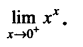Solution: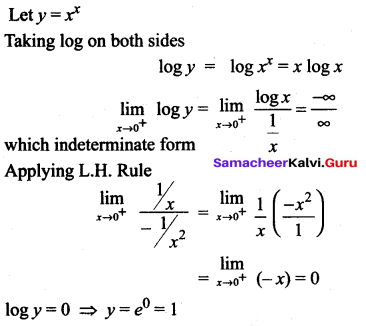Question 9.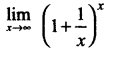Solution: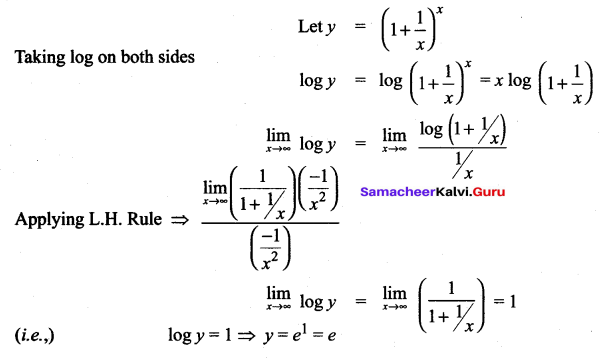Question 10.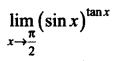Solution: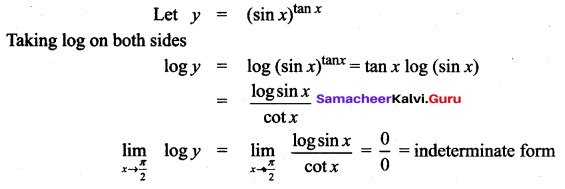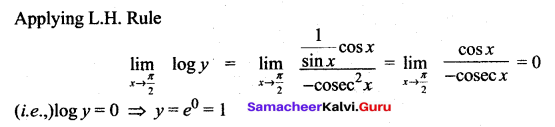Question 11.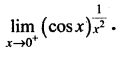Solution: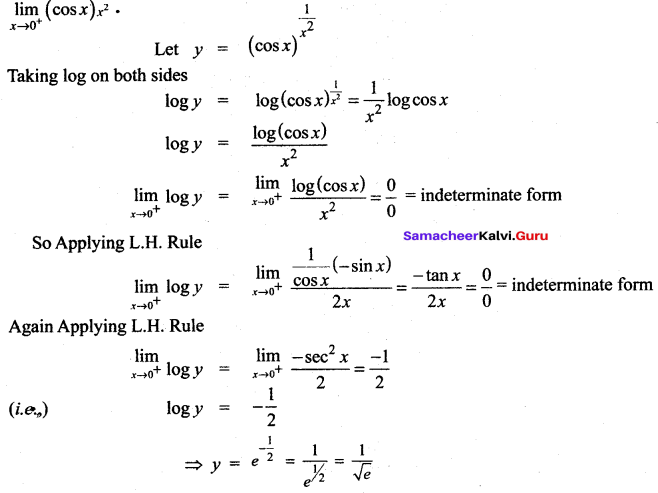Question 12.
If an initial amount A0 of money is invested at an interest rate r compounded n times a year, the value of the investment after t years is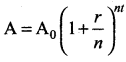. If the interest is compounded continuously, (that is as n ➝ ∞), show that the amount after t years is A = A0ert.
Solution: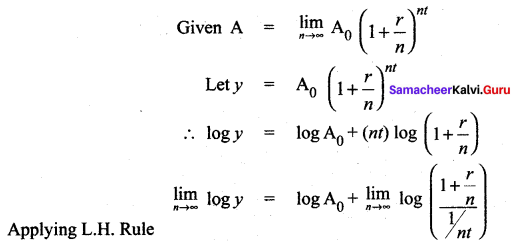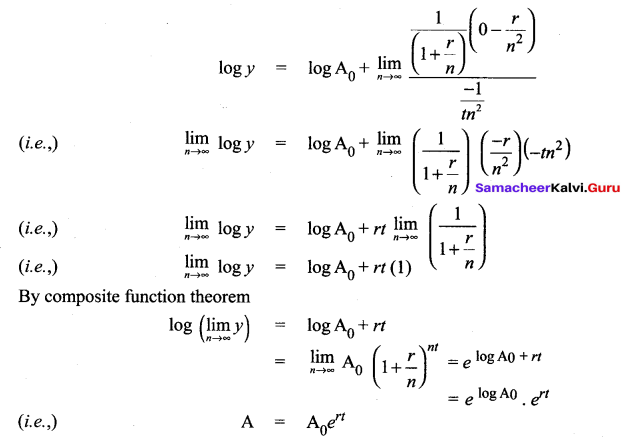### Samacheer Kalvi 12th Maths Solutions Chapter 7 Applications of Differential Calculus Ex 7.5 Additional Problems

Question 1.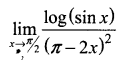Solution: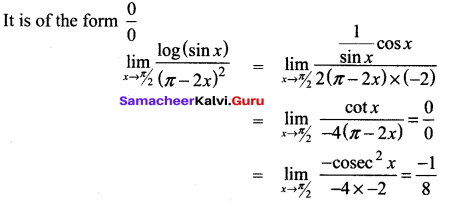Note that here l’Hopital’s rule, applied yields the result

Question 2.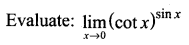Solution: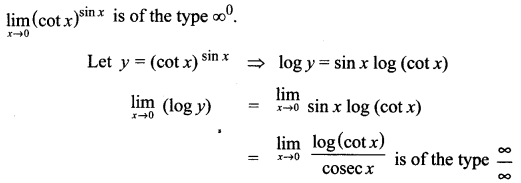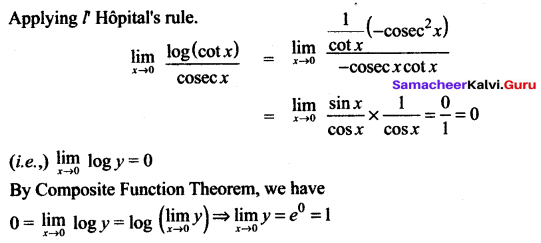Question 3.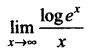Solution: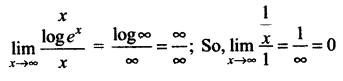Question 4.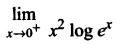Solution: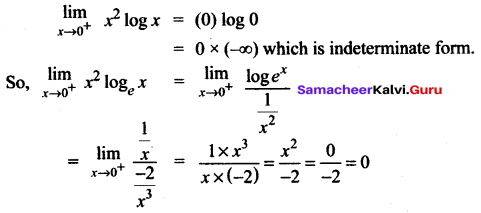Question 5.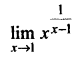Solution: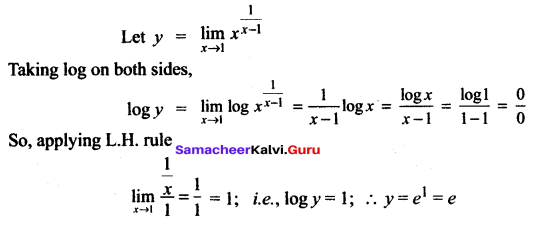Question 6.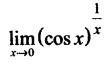Solution: